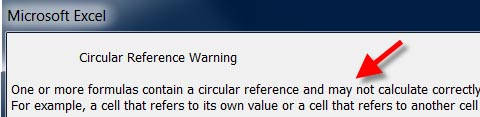# Definition: circular reference

(1) A programming conflict whereby the operation of each of two routines requires the result from the other routine. See recursion.

(2) In a spreadsheet, a conflict in which the formula computes the cell that the formula itself is located in. For example, a formula in cell A9 that sums the values of "A7 + A8 + A9" is a circular reference.